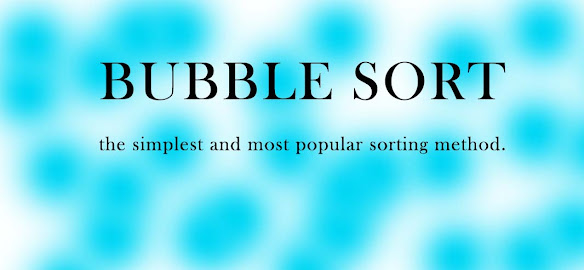# Algorithm and Example of Bubble Sort - Ishwaranand

## Bubble Sort

### Q) Explain Bubble sort algorithm with a suitable example

• Bubble sort is one of the simplest and most popular sorting methods.
• This sort is based on comparing two adjacent element,say,data[x]and data[x+1].if data[x]>data[x+1]then they are exchanged.
• To sort N elements bubble sort takes(N-1)passes.
Pass 1: Largest element is stored are Data[N] position.
Pass 2: Second largest element is stored are Data[N-1] position
Pass 3: Third largest element is stored are Data[N-2] position.
.
.
Pass N-1: Data and Dataare sorted, so that Data, Data...Data[N] are sorted.Image Source ~ Crafted With ©Ishwaranand - 2020 ~ Image by ©Ishwaranand

### Algorithm:bubble_sort (Data[],N)

• This the algorithm for bubble sort to sort the array in ascending order.
• Data[]-Array of elements
• N-size of array
• i,j-index variable
• temp-temporary variable
Step 1 : Start
Step 2 : Repeat the steps 3 and 4 for j = 1 to (N-1)
Step 3 : Repeat step 4 for j=1 to (N-1)
Step 4 : If Data [i] > Data[j+1] then
[ Exchange Data[j] & Data[j+1] ]
a) Set temp = Data[j]
b) Set Data[j] = Data[j+1]
c) Set Data[j+1] = temp
Step 5 : Stop

### Example Bubble Sort

Consider an array containing 5 elements.
Given array is A =
4223746511
Pass 1 Initial Array
4223232323
2342424242
7474746565
6565 6574 11
1111 1111 74
Pass 2 Initial Array
23232323
42424242
65656511
11111165
74747474
Pass 3 Initial Array
232323
424211
111142
656565
747474
Pass 4 Initial Array
2311
1123
4242
6565
7474
Result: Given array is sorted in 4 Passes.
1122426574